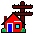# OpenVMS Source Code Demos

## BASIC_Peek_Demo.bas (test run + comments)

### Test Run

```\$ run PEEK-DEMO_102.EXE
PEEK-DEMO_102.3
===============
initializing test data
run-time array init
subscripts? (enter any number between 2 and 5) 2
-i- last subscript will be: 2
initializing test data (continue)

-i-Test-1
Long Integer= 123
Byte Peek:
2061523272 =        123
2061523273 =          0
2061523274 =          0
2061523275 =          0
hack= 123
-i-end of hack 1. Hit

-i-Test-2
Dynamic String=HELLO
Word Peek:
2061522728 =          5
Byte Peek:
2061522730 =         14
2061522731 =          2
Long Peek:
2061522732 =     280612
a=(length )  5
b=(type   )  14
c=(class  )  2
Byte Peek:
280612 =         72  = H
280613 =         69  = E
280614 =         76  = L
280615 =         76  = L
280616 =         79  = O
-i-end of hack 2. Hit

-i-Test-3
Mapped String=GOOD BYE
length  10
Byte Peek:
196624 =         71  = G
196625 =         79  = O
196626 =         79  = O
196627 =         68  = D
196628 =         32  =
196629 =         66  = B
196630 =         89  = Y
196631 =         69  = E
196632 =         32  =
196633 =         32  =
196634 =          0  = .
196635 =          0  = .
-i-end of hack 3. Hit

-i-Test-4
fs array (fixed length strings - no descriptors)
array data
0  FS0
1  FS1
2  FS2
declared max size: 5
declared max subs: 2
length  5
Byte Peek:
196608 =         70  = F
196609 =         83  = S
196610 =         48  = 0
196611 =         32  =
196612 =         32  =
196613 =         70  = F
196614 =         83  = S
196615 =         49  = 1
196616 =         32  =
196617 =         32  =
196618 =         70  = F
196619 =         83  = S
-i-end of hack 4. Hit

-i-Test-5
vs array (compile-time - variable length strings - descriptors)
array data
0  VSC0
1  VSC1
2  VSC2
Word Peek:
2061522872 =          4
Byte Peek:
2061522874 =         14
2061522875 =          2
Long Peek:
2061522876 =     280660
a=(length )  4
b=(type   )  14
c=(class  )  2
Byte Peek:
280660 =         86  = V
280661 =         83  = S
280662 =         67  = C
280663 =         48  = 0
hit  to continue
Word Peek:
2061522880 =          4
Byte Peek:
2061522882 =         14
2061522883 =          2
Long Peek:
2061522884 =     280676
a=(length )  4
b=(type   )  14
c=(class  )  2
Byte Peek:
280676 =         86  = V
280677 =         83  = S
280678 =         67  = C
280679 =         49  = 1
-i-end of hack 5. Hit

-i-Test-6 <<<
vs array (run-time - variable length strings - descriptors)
array data
0  VSR0
1  VSR1
2  VSR2
Word Peek:
344464 =          4
Byte Peek:
344466 =         14
344467 =          2
Long Peek:
344468 =     280780
a=(length )  4
b=(type   )  14
c=(class  )  2
-i-Test-6a <<<
Byte Peek:
280780 =         86  = V
280781 =         83  = S
280782 =         82  = R
280783 =         48  = 0
hit  to continue
-i-Test-6b <<<
Byte Peek:
280796 =         86  = V
280797 =         83  = S
280798 =         82  = R
280799 =         49  = 1
-i-Test-6c <<<
Byte Peek:
280796 =         86  = V
280797 =         83  = S
280798 =         82  = R
280799 =         49  = 1
-i-end of hack 6. Hit
\$
```

Back in the 1990s, the computer industry was transitioning from CISC technology (like VAX) to RISC technology (like Alpha). There were numerous reasons for this shift but the biggest one involved resources lost while servicing interrupts. Consider the following example from the VAX world.
1. CISC instructions are decoded into microcode for execution (since memory was expensive, the best CISC architectures attempted to do as much microcode as possible for every CISC instruction)

2. Some CISC instructions could consume several hundred milliseconds of CPU resources (right now I am thinking about the POLYF instruction)

3. Now some external event occurs (like an interrupt or virtual page fault exception). Since there was no way to save a partially executed instruction, all the work done up to the event is lost. This means that the interrupted instruction must be restarted rather than resumed.

4. Engineers could have gone to great lengths to save a partially executed CISC instruction (by saving the microcode), but with memory costs dropping exponentially, it was easier to modify compilers so they compiled directly to microcode.  Programs would be larger, but now the equivalent of a CISC instruction could be interrupted, then resumed.

Once engineers had transitioned from CISC to RISC, other technologies would now be possible. Like:

• prefetching instructions into the CPU (even if it only loads data into the high-speed CPU cache)
• branch prediction
• speculative execution of branches
• out-of-order execution

For all these technologies to work optimally, the CPU needs to make good guesses while minimizing the unnecessary generation of page faults. Much of this happens via techniques like: inlining subroutines, loop unrolling, doing as much compile-time work as possible, inferring then inlining constants etc.

###

In the poke example listed above, I was surprised to see that the size of fixed-sized arrays and fixed-length strings was encoded as inline constants.  In the VAX days you would have found a properly populated array descriptor pointing to string descriptors (fixed or dynamic).Back to Home
Neil Rieck